Get instant live expert help with Excel or Google Sheets“My Excelchat expert helped me in less than 20 minutes, saving me what would have been 5 hours of work!”

#### Post your problem and you’ll get Expert help in seconds.

Your message must be at least 40 characters
Our professional Expert are available now. Your privacy is guaranteed.

# How to Solve the System of Equations in Excel

In mathematical terms, the system of equation is set of two or more equations having the same set of unknown variables like x, y, z where we need to find the values of unknown variables to solve these equations. To solve the system of equations, we can utilize functions and the equation solver tool.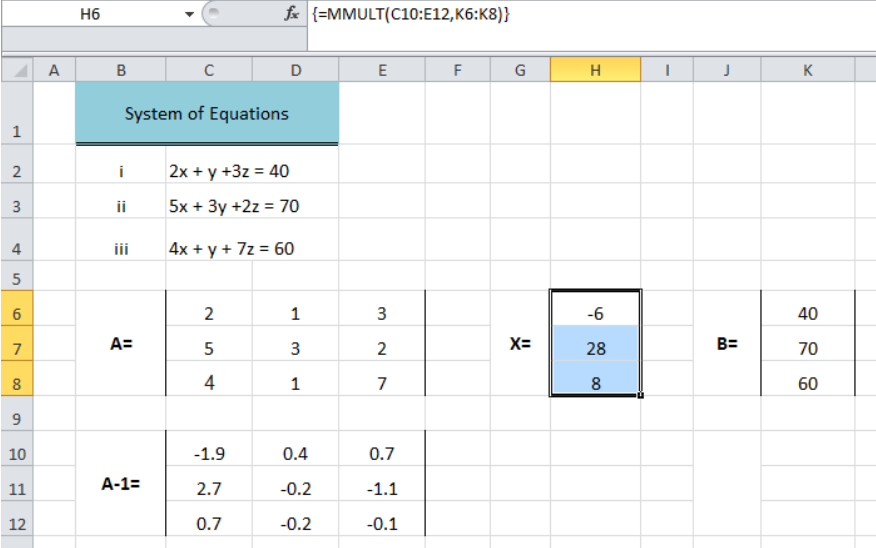Figure 1. How to Solve the System of Equations

## Using Excel Functions

We can solve the system of equations by using MINVERSE and MMULT mathematical functions. Suppose we have three equations in our system of equations in our example. To solve equations using functions we need to set up the data as follows;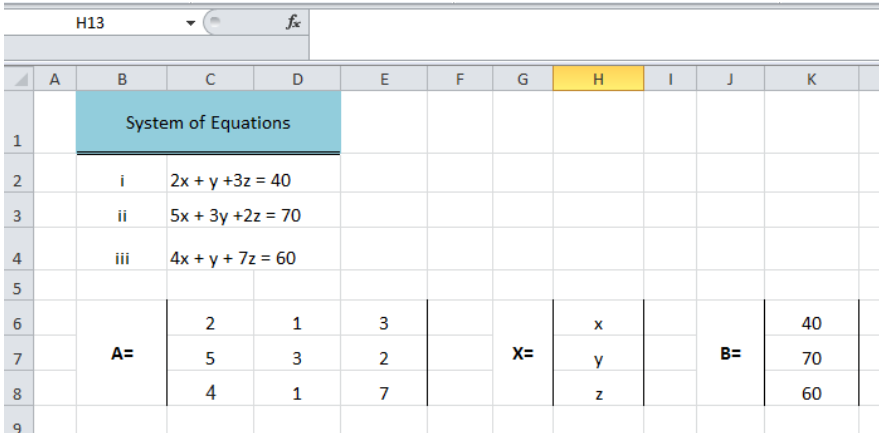Figure 2. Setting Up Data of System of Equations

In matrix notation form we can write it as;

`AX= B`

And to find the value of unknown variables X we need to find the inverse of matrix A (A-1) and multiply it with matrix B, such as;

`X= A-1B`

To find the inverse of matrix A (A-1) we need to use the MINVERSE function as follows;

• Select the range of cells as per the matrix A layout such as C10: E12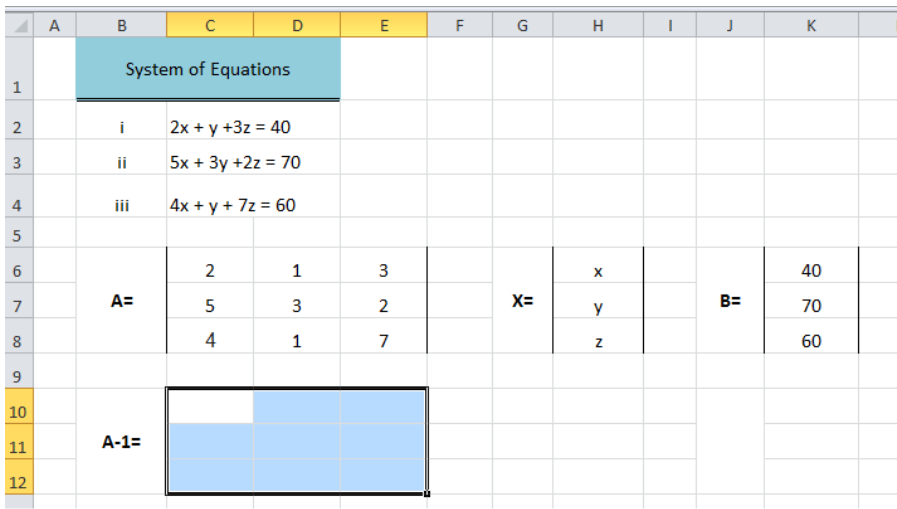Figure 3. Selecting Range of Cells

• Go to the formula bar and insert the MINVERSE formulas as `=MINVERSE(C6: E8)` and press Ctrl + Shift + Enter instead of pressing Enter key to make it an array formula to return an array of value as the inverse of matrix A.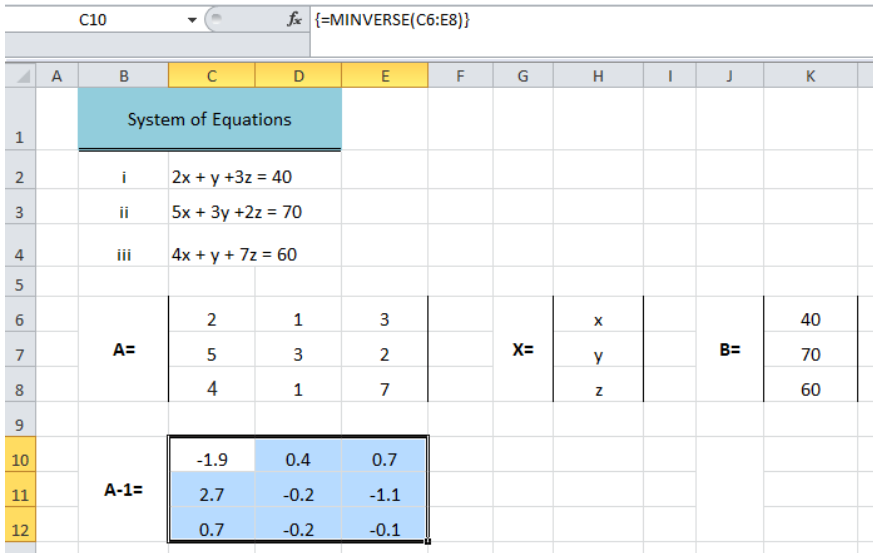Figure 4. Finding Inverse of Matrix A

Now we need to find the values of unknown variables in matrix X (x, y, z) in column H to solve the system of equations using Excel MMULT function as follows;

• Select the range of cells H6: H8 of matrix X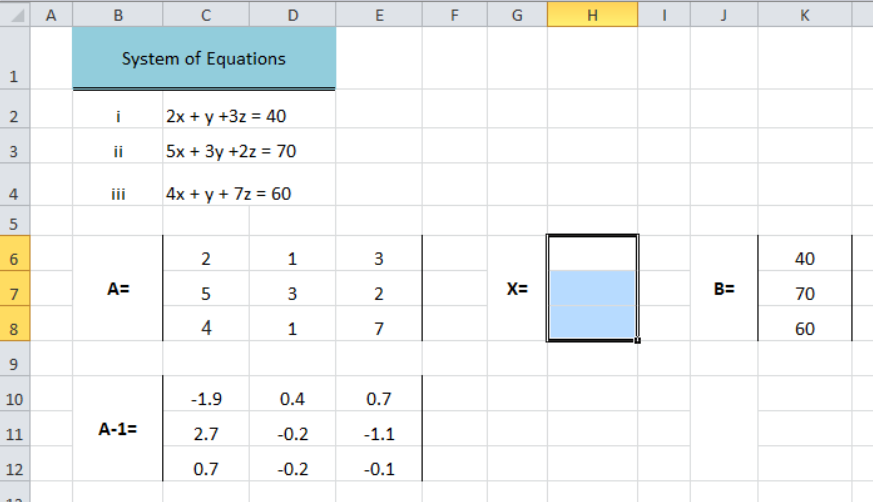Figure 5. Selecting Range of Cells of Matrix X

• In the formula bar, insert the MMULT formula as `=MMULT(C10: E12, K6: K8)` to return the product of two matrix A-1 (inverse of Matrix A) and B by using its arrays and press Ctrl + Shift + Enter to make it an array formula. This formula returns the values of unknown variables and solves the system of equations.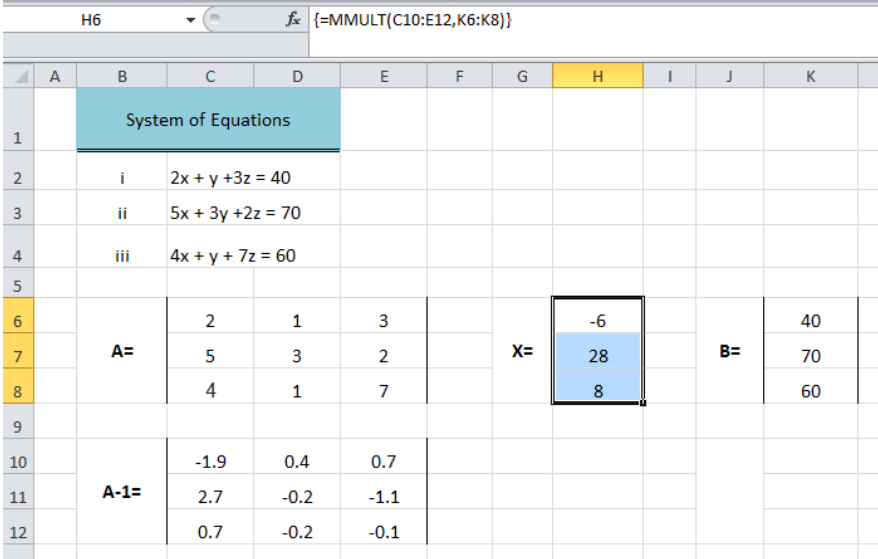Figure 6. Solve System of Equations

Another approach to solve the system of equations is Excel Solver Add-in. We need to install this add-in from available add-ins from Options > Add-ins. For Excel Solver we need to set up the data of the system of equations as follows;

• For matrix A we need to enter the formula for each of the equations in column C as shown.
• Insert the values of matrix B in column D.
• Leave the cells of matrix X blank in column E to return the values of unknown variables x, y, z.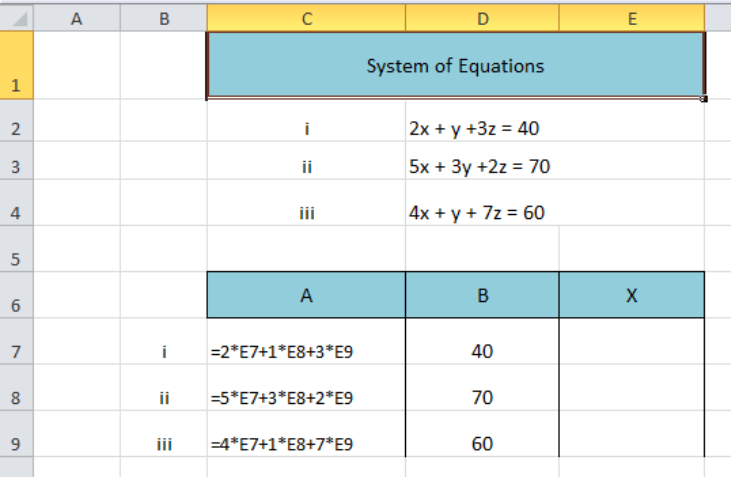Figure 7. Setting Up Data of Equations for Solver

• Go to Data tab, from Analysis group select the Solver to launch.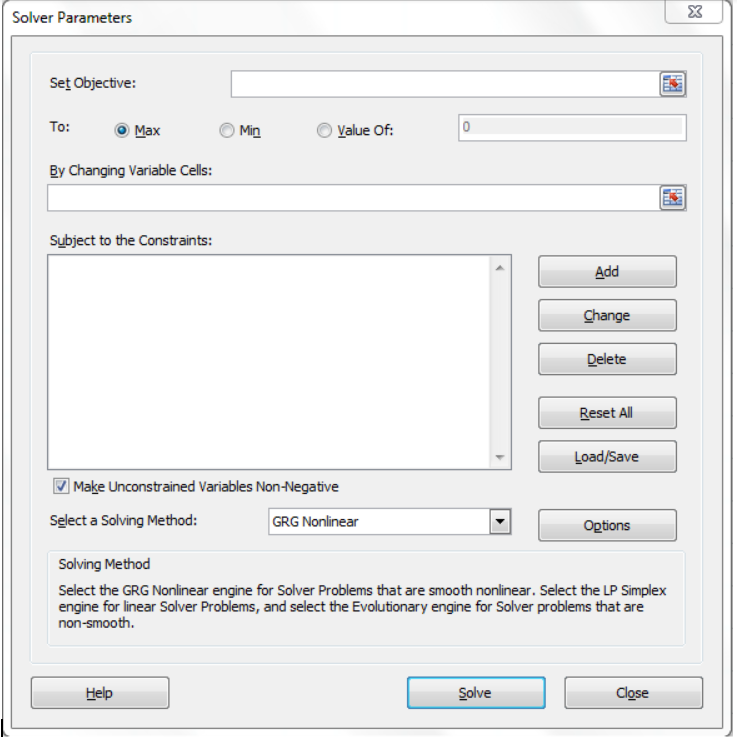Figure 8. Solver Parameters Window

• In the Set Objective box insert the cell reference C7, the first cell of matrix A where we have set the formula for the first equation of the system.
• Select the objective as Max.
• Insert the range of cell references E7: E9 of matrix X in the By Changing Variable Cells box.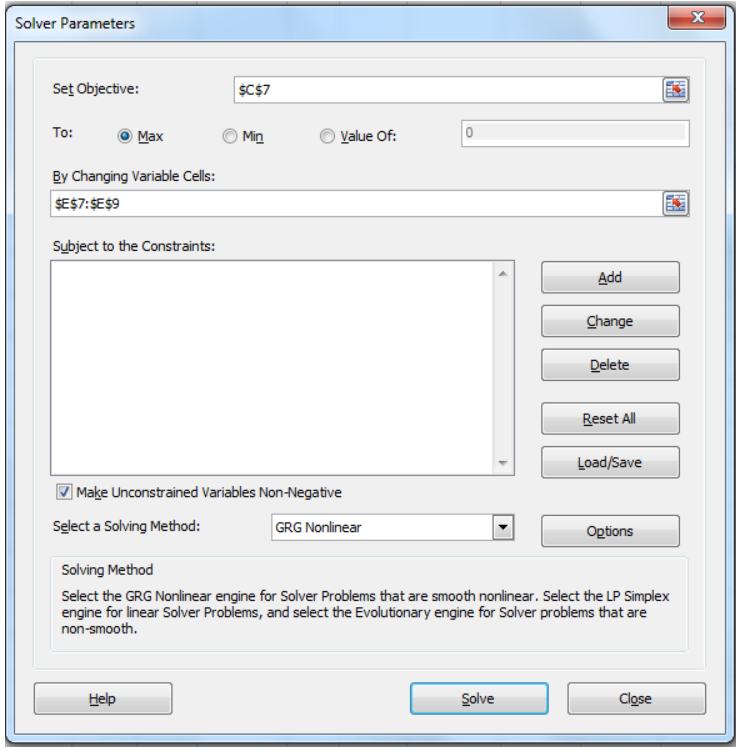Figure 9. Setting Up Solver Parameters

• In the Subject to Constraints box, we need to add the constraints for each of the equations by clicking on Add button.
• In the Add Constraints window, we need to enter the constraints for all equations one by one by pressing Add button. Press the OK button when we are done with adding all the constraints.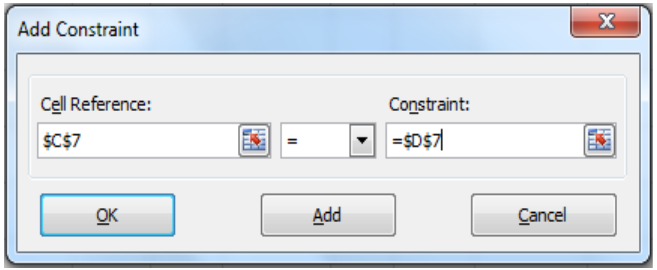Figure 10. Adding Constraints in Solver

• After adding all the constraints, we need to unselect the Make Unconstrained Variables Non-negative checkbox below the constraints box.
• Press the Solve button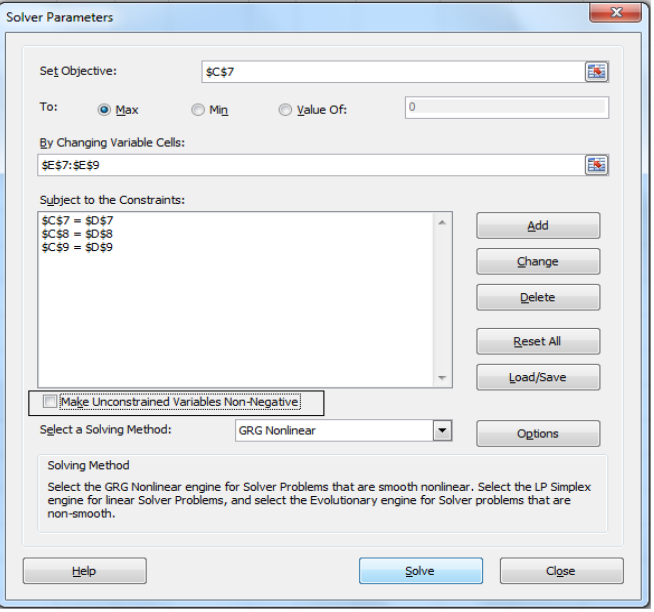Figure 11. Solving Equations

• On Solver Results window, select Keep Solver Solutions and press OK button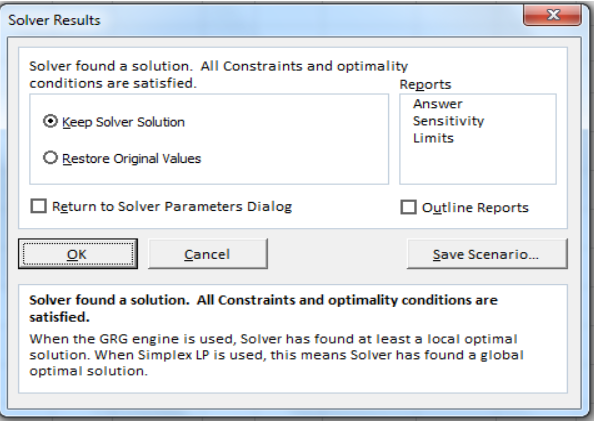Figure 12. Solver Results

• Solver returns the values of unknown variables for matrix X in column E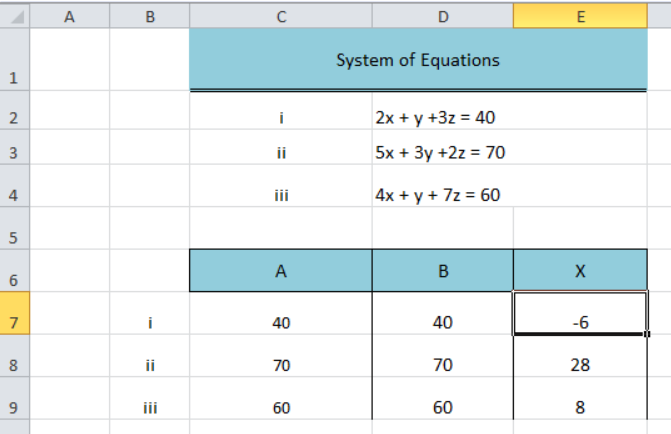Figure 13. Equation Solver

## Instant Connection to an Expert through our Excelchat Service

Most of the time, the problem you will need to solve will be more complex than a simple application of a formula or function. If you want to save hours of research and frustration, try our live Excelchat service! Our Excel Experts are available 24/7 to answer any Excel question you may have. We guarantee a connection within 30 seconds and a customized solution within 20 minutes.